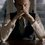# Harmonic series sum

I was wondering on a problem in Alan Tucker's Combinatorics when I noticed this and I wanted to know if it is true(Though I have checked on Wolfram Alpha and it is quite true).

The generating function for ${a}_{r} = \frac{1}{r}$ is of course $-log(1-x)$.

Therefore, $h(x) = -log(1-x)$

Hence, $h*(x) = \frac{-log(1-x)}{1-x}$ , where $h * (x)$ is the generating function for the sums of ith coefficient of $h(x)$.

As a result, the co-efficient of ${x}^{n}$ in $h*(x)$ is equal to $1 + \frac{1}{2} + \frac{1}{3}+.... + \frac{1}{n}$.

If this is true, is there any way to find the coefficient of ${x}^{n}$ in $\frac{-log(1-x)}{1-x}$?Note by Kartik Sharma
6 years, 4 months ago

This discussion board is a place to discuss our Daily Challenges and the math and science related to those challenges. Explanations are more than just a solution — they should explain the steps and thinking strategies that you used to obtain the solution. Comments should further the discussion of math and science.

When posting on Brilliant:

• Use the emojis to react to an explanation, whether you're congratulating a job well done , or just really confused .
• Ask specific questions about the challenge or the steps in somebody's explanation. Well-posed questions can add a lot to the discussion, but posting "I don't understand!" doesn't help anyone.
• Try to contribute something new to the discussion, whether it is an extension, generalization or other idea related to the challenge.
• Stay on topic — we're all here to learn more about math and science, not to hear about your favorite get-rich-quick scheme or current world events.

MarkdownAppears as
*italics* or _italics_ italics
**bold** or __bold__ bold
- bulleted- list
• bulleted
• list
1. numbered2. list
1. numbered
2. list
Note: you must add a full line of space before and after lists for them to show up correctly
paragraph 1paragraph 2

paragraph 1

paragraph 2

[example link](https://brilliant.org)example link
> This is a quote
This is a quote
    # I indented these lines
# 4 spaces, and now they show
# up as a code block.

print "hello world"
# I indented these lines
# 4 spaces, and now they show
# up as a code block.

print "hello world"
MathAppears as
Remember to wrap math in $$ ... $$ or $ ... $ to ensure proper formatting.
2 \times 3 $2 \times 3$
2^{34} $2^{34}$
a_{i-1} $a_{i-1}$
\frac{2}{3} $\frac{2}{3}$
\sqrt{2} $\sqrt{2}$
\sum_{i=1}^3 $\sum_{i=1}^3$
\sin \theta $\sin \theta$
\boxed{123} $\boxed{123}$

## Comments

Sort by:

Top Newest

You mean a closed form? I'm afraid I don't know of one (it probably does not exist). However, the asymptotic limit is $\gamma+\ln n$, where $\displaystyle \gamma = \int_{1}^{\infty} \frac{1}{\lfloor x \rfloor}-\frac{1}{x} dx$ is the Euler-Mascheroni constant.

- 6 years, 4 months ago

Log in to reply

Are you sure this is true? *I haven't heard of anything like that. BTW, try finding the coefficient of the $h(x)$ too(with proof, if you can). Thanks for that info!

- 6 years, 4 months ago

Log in to reply

By $h * (x)$, do you mean $h' (x)$?

Staff - 6 years, 4 months ago

Log in to reply

No sir, by h*(x), I meant the generating function of the sums of the ${a}_{r}$s.

- 6 years, 4 months ago

Log in to reply

Thanks. I added it in to clarify what that terminology is.

Usually for such Maclaurin expansions, you simply multiply the different parts together.

Staff - 6 years, 4 months ago

Log in to reply

- 6 years, 4 months ago

Log in to reply

×

Problem Loading...

Note Loading...

Set Loading...